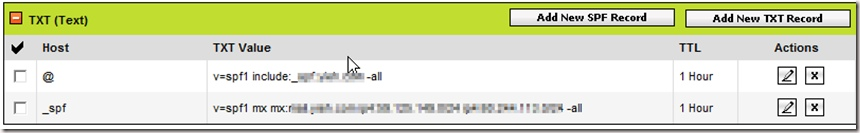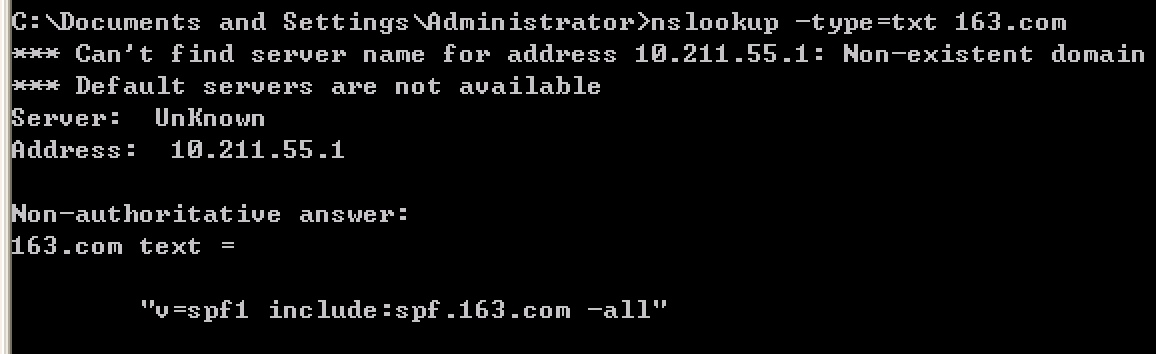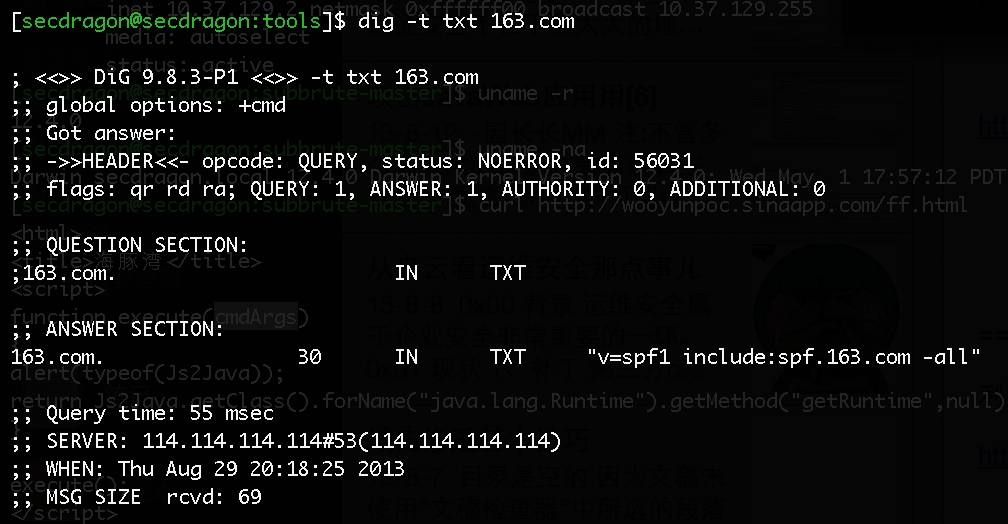# 原文地址:http://drops.wooyun.org/papers/534

## 0x01 细节

SMTP协议中,允许发件人伪造绝大多数的发件人特征信息。

http://emkei.cz/

## 0x02 防御

SPF(或是Sender ID)是Sender Policy Framework的缩写。

``````Received-SPF: pass (google.com: domain of [email protected]/* <![CDATA[ */!function(t,e,r,n,c,a,p){try{t=document.currentScript||function(){for(t=document.getElementsByTagName('script'),e=t.length;e--;)if(t[e].getAttribute('data-cfhash'))return t[e]}();if(t&&(c=t.previousSibling)){p=t.parentNode;if(a=c.getAttribute('data-cfemail')){for(e='',r='0x'+a.substr(0,2)|0,n=2;a.length-n;n+=2)e+='%'+('0'+('0x'+a.substr(n,2)^r).toString(16)).slice(-2);p.replaceChild(document.createTextNode(decodeURIComponent(e)),c)}p.removeChild(t)}}catch(u){}}()/* ]]> */_domain.com designates 72.47.192.112 as permitted sender) client-ip=72.47.192.112;
Authentication-Results: mx.google.com; spf=pass (google.com: domain of [email protected]/* <![CDATA[ */!function(t,e,r,n,c,a,p){try{t=document.currentScript||function(){for(t=document.getElementsByTagName('script'),e=t.length;e--;)if(t[e].getAttribute('data-cfhash'))return t[e]}();if(t&&(c=t.previousSibling)){p=t.parentNode;if(a=c.getAttribute('data-cfemail')){for(e='',r='0x'+a.substr(0,2)|0,n=2;a.length-n;n+=2)e+='%'+('0'+('0x'+a.substr(n,2)^r).toString(16)).slice(-2);p.replaceChild(document.createTextNode(decodeURIComponent(e)),c)}p.removeChild(t)}}catch(u){}}()/* ]]> */_domain.com designates 72.47.192.112 as permitted sender) [email protected]/* <![CDATA[ */!function(t,e,r,n,c,a,p){try{t=document.currentScript||function(){for(t=document.getElementsByTagName('script'),e=t.length;e--;)if(t[e].getAttribute('data-cfhash'))return t[e]}();if(t&&(c=t.previousSibling)){p=t.parentNode;if(a=c.getAttribute('data-cfemail')){for(e='',r='0x'+a.substr(0,2)|0,n=2;a.length-n;n+=2)e+='%'+('0'+('0x'+a.substr(n,2)^r).toString(16)).slice(-2);p.replaceChild(document.createTextNode(decodeURIComponent(e)),c)}p.removeChild(t)}}catch(u){}}()/* ]]> */_domain.com
``````

http://www.microsoft.com/mscorp/safety/content/technologies/senderid/wizard/default.aspx

http://old.openspf.org/wizard.html``````a 你域名的A记录，一般选择yes，因为他有可能发出邮件，比如我上面提到的Wordpress的回信。
mx 一般也是yes，MX服务器会有退信等。
ptr 选择no，官方建议的。
ip4： 你还有没有其他的ip发信？可能你的smtp服务器是独立出来的，那么就填入你的IP地址或者网段。
~all: 意思是除了上面的，其他的都不认可。当然是yes了。
``````

Windows下进入DOS模式后用以下命令

``````nslookup -type=txt 域名
``````Unix操作系统下用：

``````# dig -t txt 域名
``````